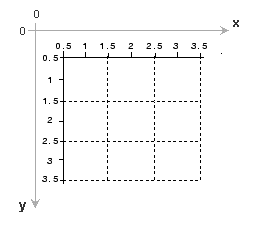## Image Coordinate Systems

As described in Images in MATLAB, MATLAB® stores most images as two-dimensional arrays (i.e., matrices), in which each element of the matrix corresponds to a single pixel in the displayed image. To access locations in images, the Image Processing Toolbox™ uses several different image coordinate systems as conventions for representing images as arrays.

• Pixel Indices—Because images are arrays, you can use standard MATLAB indexing.

• Spatial Coordinates—You can consider locations in images as positions on a plane using Cartesian coordinates.

### Pixel Indices

Often, the most convenient method for expressing locations in an image is to use pixel indices. The image is treated as a grid of discrete elements, ordered from top to bottom, and left to right, as illustrated by the following figure.

Pixel IndicesFor pixel indices, the row increases downward, while the column increases to the right. Pixel indices are integer values, and range from 1 to the length of the row or column.

There is a one-to-one correspondence between pixel indices and subscripts for the first two matrix dimensions in MATLAB. For example, the data for the pixel in the fifth row, second column is stored in the matrix element (5,2). You use normal MATLAB matrix subscripting to access values of individual pixels. For example, the MATLAB code

`I(2,15)`

returns the value of the pixel at row 2, column 15 of the image `I`. Similarly, the MATLAB code

`RGB(2,15,:) `

returns the `R`, `G`, `B` values of the pixel at row 2, column 15 of the image `RGB`.

The correspondence between pixel indices and subscripts for the first two matrix dimensions in MATLAB makes the relationship between an image's data matrix and the way the image is displayed easy to understand.

### Spatial Coordinates

Another method for expressing locations in an image is to use a system of continuously varying coordinates rather than discrete indices. This lets you consider an image as covering a square patch, for example. In a spatial coordinate system like this, locations in an image are positions on a plane, and they are described in terms of x and y (not row and column as in the pixel indexing system). From this Cartesian perspective, an (x,y) location such as (3.2,5.3) is meaningful, and is distinct from pixel (5,3).

The Image Processing Toolbox defines two types of spatial coordinate systems

#### Intrinsic Coordinates

By default, the toolbox uses a spatial coordinate system for an image that corresponds to the image’s pixel indices. It is called the intrinsic coordinate system and is illustrated in the following figure. Notice that y increases downward, because this orientation is consistent with the way in which digital images are typically viewed.

Intrinsic Coordinate SystemThe intrinsic coordinates (x,y) of the center point of any pixel are identical to the column and row indices for that pixel. For example, the center point of the pixel in row 5, column 3 has spatial coordinates x = 3.0, y = 5.0. This correspondence simplifies many toolbox functions considerably. Be aware, however, that the order of coordinate specification (3.0,5.0) is reversed in intrinsic coordinates relative to pixel indices (5,3).

Several functions primarily work with spatial coordinates rather than pixel indices, but as long as you are using the default spatial coordinate system (intrinsic coordinates), you can specify locations in terms of their columns (x) and rows (y).

When looking at the intrinsic coordinate system, note that the upper left corner of the image is located at (0.5,0.5), not at (0,0), and the lower right corner of the image is located at (`numCols` + 0.5, `numRows` + 0.5), where `numCols` and `numRows` are the number of rows and columns in the image. In contrast, the upper left pixel is pixel (1,1) and the lower right pixel is pixel (`numRows`, `numCols`). The center of the upper left pixel is (1.0, 1.0) and the center of the lower right pixel is (`numCols`, `numRows`). In fact, the center coordinates of every pixel are integer valued. The center of the pixel with indices (r, c) — where r and c are integers by definition — falls at the point x = c, y = r in the intrinsic coordinate system.

#### World Coordinates

In some situations, you might want to use a world coordinate system (also called a nondefault spatial coordinate system). For example, you could shift the origin by specifying that the upper left corner of an image is the point (19.0,7.5), rather than (0.5,0.5). Or, you might want to specify a coordinate system in which every pixel covers a 5-by-5 meter patch on the ground.

There are several ways to define a world coordinate system: